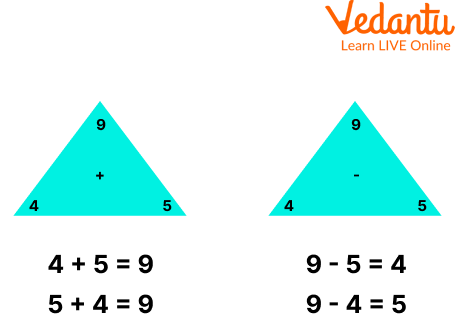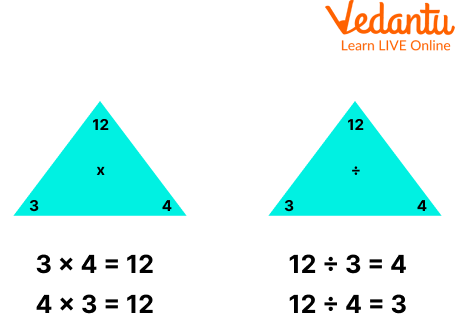Courses
Courses for Kids
Free study material
Offline Centres
More

# What is Fact Family in Maths?Last updated date: 05th Dec 2023
Total views: 98.7k
Views today: 2.98k## Introduction to Fact Family Maths

The idea of a fact family or families of facts is one of the more frequent topics introduced in first-grade maths. Fact family maths helps your kids discover the relationships between numbers and the reasoning behind addition and subtraction. An interesting way to organise the study of maths facts is through Fact Family. A group of mathematical operations or equations produced using the same set of numbers is referred to as a fact family multiplication. The relationship between the three numbers is demonstrated by the fact of family. There are four addition and subtraction statements made using three numbers in an addition and subtraction fact family.

## What is a Fact Family?

Fact family definition states that a series of equations or calculations involving the same three integers. Fact family's definition begins with two numbers joining together, just like a family. Say, for example, that 1 and 2 eventually cross paths. Then, they combine to form 3. There seems to be their initial family picture: 1 + 2 = 3.

Furthermore, we can change the numbers we're adding and still come up with the same total. Therefore, 1, 2, and 3 might alternatively be arranged as follows: 2 + 1 = 3.

Similarly, numbers might have various relationships with one another at certain times, just like people in families have. A subtraction sentence can have the numbers 3, 2, and 1.

We may begin by subtracting 2 from 3 using the same three fact family members, giving us this image of the three numbers.

3 - 2 = 1

Of course, we could also reduce 3 by 1 to get 2: 3 - 1 = 2.

## Fact Families Multiplication and Division

A collection of connected arithmetic facts using the same three integers is referred to as a fact family multiplication. Division, the inverse or opposite operation of multiplication, can likewise be included in the fact family of division. The fact family would include four equations, for instance, if 2, 5, and 10 were used: 2 x 5 = 10, 5 x 2 = 10, 10 ÷ 5 = 2.

Fact family multiplication continues to improve your child's understanding of how numbers relate to one another in maths since students may have used fact families while learning addition and subtraction. The commutative property of fact family multiplication, which states that 2 x 5 is the same as 5 x 2, is highlighted through fact families.

## Fact Family Examples

Some fact family examples are assigned to the students that are based on the fact family definition:

Example 1: Use the numbers 9, 4, and 5 to build an addition and subtraction, fact family.

Solution: We know that four addition and subtraction statements were produced using 9, 4, and 5 and form an addition and subtraction fact family as shown below:Example 2: Example 1: Use the numbers 12, 4, and 3 to build a multiplication and division fact family.

Solution: We know that four multiplication and division statements were produced using 12, 4, and 3 and form a fact family multiplication and division as shown below:Fact families multiplication and division

## Practice Problems

Q1. Which mathematical operation is NOT a member of the group of fact family you can construct using the numbers 8, 5, and 13?

(a) 8 + 5

(b) $8 \times 5$

(c) 13 - 5

(d) 13 - 8

Ans. (b) $8 \times 5$

Q2. Which digit from this 9 +_ _ _ = 18 family of facts is missing?

(a) 8

(b) 9

(c) 27

(d) 6

### Ans. (b) 9

Q3. What comes next in the addition of a family of 8 and 40?

Ans. 48

## Summary

So far we have discussed what a fact family is. Fact family definition is a group of three numbers that together make four mathematical operations utilising one operator and its inverse. Because addition and multiplication operations are commutative—a fancy way of saying that the order of their arguments doesn't matter, families are a result. The fact of family demonstrates the connections between the numbers. This article explains the addition, subtraction, multiplication and division fact families with fact family examples. A strong understanding of four basic maths operations and their connections will be developed by solving the practice problems provided in this article progressively.

## FAQs on What is Fact Family in Maths?

1. At which age should kids learn multiplication tables?

After a child has learned the fundamentals of addition and subtraction, is familiar with arrays and can count by twos and fives, which is often by the age of nine, they can start learning their multiplication tables. Since every child is different, some might be able to learn multiplication tables earlier than this. Knowing whether your child is prepared to move from addition and subtraction to learning multiplication comes from working with them at home and having them practise arithmetic concepts.

2. Where do fact families come into play?

Mathematical fact families are a useful tool for everyday life, helping you to organise groups and determine how many distinct types of arrangements are possible with three numbers. Fact families can also be used to show how numbers relate to one another. They provide a crucial basis for harder arithmetic problems like algebraic equations. Fact families can be used to help kids remember the fundamental addition and subtraction facts as well as to reinforce or learn the relationship between addition and subtraction.

3. What are the four fundamental operations in mathematics?

The four basic operations of arithmetic can be used to add, subtract, multiply, or divide two or more items. They involve the study of numbers and the order of operations, which are important in all other areas of mathematics like algebra, data processing, and geometry. Without applying the laws of arithmetic operations, we are unable to solve the issue. The four fundamental rules of mathematics are addition, subtraction, multiplication, and division.